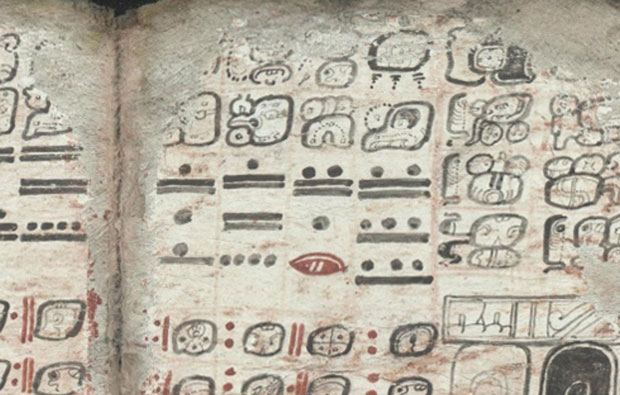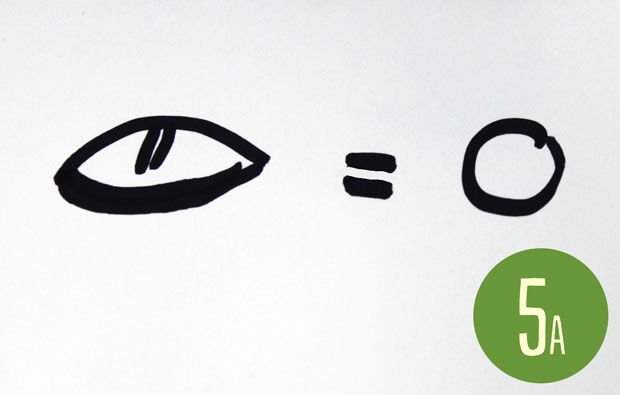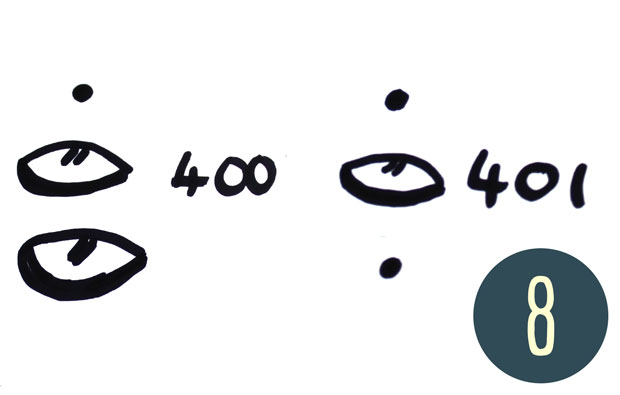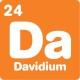# What's new## Maya numbers

By David, 7 March 2017 ActivityWant to learn how to read these ancient numbers?

The numbers that we use are not the only way to write numbers. You might know how to count with tally marks, or read Roman numerals. But there are plenty of other ways to write numbers. This one comes from the Maya people.

### You will need

• Pen and paper

### What to doTo write numbers up to 20, draw dots for ones and horizontal lines for fives.

1. Let’s start at the start. To write a 1, simply draw a dot!
2. The numbers 2, 3 and 4 are equally easy – just put the right number of dots, sideways in a row.
3. The number 5 is a horizontal line. To make 6 to 9, put dots above the line.
4. To make 10, put a second horizontal line below the first. You can add dots to make the numbers 11–14, a third line to make 15, and more dots to make 16–19.To write zero, draw a shell shape.

5. Here’s where it gets interesting! Our number system is based on 10, which is written as a 1 and a 0. Maya numbers are based around the number 20, so in Maya numbers, 20 is a 1 and a 0. So write a dot for the 1 and leave a gap below it. Then below the gap, draw a clamshell – the Mayan symbol for zero.
6. To write numbers from 21–39, think of them as 20 plus something. For example, 31 is 20 + 11, so draw a dot, then a gap, then a dot and two lines (11 in Mayan!).A Maya 20: the shell is in the ones place, so the dot is in the twenties place!

7. To write numbers up to 399, think of the number as ‘some 20s + something’. For example, 165 is eight 20s, plus five. To write this, draw three dots and a line (8), then a gap, and finally a line (5).
8. 399 is nineteen 20s plus 19, which makes it the largest number you can write with two Mayan ‘digits’. To write 400, think of it as twenty 20s. So 400 is written as a dot, a gap, a clamshell, a gap and a second clamshell.The single dot is in the twenties place, and the 11 is in the ones place, so this number is 31.

### What’s happening?

The remarkable Maya civilisation lasted for thousands of years, until they were conquered by the Spanish more than 300 years ago. They invented a full written language, and also had an advanced number system.To write bigger numbers, you can add a place on top of the ones and twenties. In these two numbers, the dot on top is in the 400s place.

Although it looks basic – almost like tally marks – the Maya number system is very sophisticated. It is one of the oldest number systems with a zero. This symbol dates back to at least 36 BCE, when the Roman Empire was still using Roman numerals.

You might have some difficulty using Maya numbers, because they are based around the number 20 rather than the number 10. But there’s actually no reason why our 10-based system is easier to use. You only find our numbers easier because that’s what you were taught!

If you’re after more hands-on activities for kids, subscribe to Double Helix magazine!Categories:

## Similar posts

1.I do it a bit differently to that when I do the set out of the symbol

1.Hi Hi,
Yup, there are probably many different ways to write these numbers – they were used over a large area for over a thousand years.

If you look at old textbooks, such as Fibonacci’s Liber Abaci, you’ll notice some of the numerals are completely the wrong shape! our 1234567890 shapes are only about 500 years old!

2.1.Hi Don’t,
Just like with our number system, you can keep counting by adding more digits to the front of the number. Only with Mayan numbers, the next digit goes on top!
This activity explores the ones, twenties and four hundreds digits. If you put a fourth digit on top, it’s in the eight thousands place- so dot, shell, shell, shell is eight thousand!

3.I have a theory on how the Mayan might have used their fingers to count to 20. I made a video. What do you think?

https://youtu.be/GQTTM_ZMmvI

4.What is the largest number that can be counted to, in a vigesimal
system with four places

1.It’s probably the value of the smallest 5 digit number, minus 1!

5.COOL COOL I did not know that

6.Hi… I have a question;
What is the largest number that can be counted to, in a vigesimal (base-20) system with four places? Please write the answer using numeric digits only.

1.The largest four digit number is just a bit smaller than the smallest five digit number. So work that out, and count backwards to get your answer!

7.How would you write 2020?

1.Hi Jorge,
We’ll need to write 2020 in terms of 400, 20 and 1:
2020 = 5 x 400 + 1 x 20 + 0 x 1
then we convert to symbols:
horizontal line = 5, dot = 1, shell = 0
and that’s the answer – horizontal line, gap, dot, gap, shell.

8.why don’t you have twelve and eight

9.Do you have copyable Mayan numerals? Like ones I can copy and paste of a document, that aren’t a image.

1.you can grab some images of numerals on wikipedia:
https://en.wikipedia.org/wiki/Maya_numerals

if you have the right font installed (I don’t know which one you need sadly), you could use these unicode characters:
https://en.wikipedia.org/wiki/Mayan_Numerals_(Unicode_block)

hope this helps!

10.1.